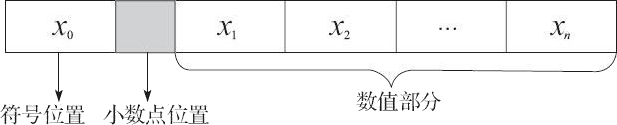## C语言除法算法和取模运算的实现（多种算法，多种思路）

• 内容
• 评论
• 相关

```if (x/y>z)
{
// ...
}```

```if (((y>0)&&(x>y*z))||((y<0)&&(x<y*z)))
{
// ...
}```

```double x=a/b/c;
double y=a/b+c/b;```

```double x=a/(b*c);
double y=(a+c)/b;```

a=a%8;

a=a&7;

x=(x+y)%z;

```x+=y;
while(x>=z)
{
x-=z;
}```

## 用倒数相乘来实现除法运算

x/y=x*(1/y)

10/5=10*(1/5)=10*0.2=2

```#include <stdio.h>
int main(void)
{
int x = 3/2;
float y = 3.0/2.0;
printf("3/2 = %d\r\n3.0/2.0 = %1.1f\n",x,y);
return 0;
}```

3/2 = 1
3.0/2.0 = 1.50条评论